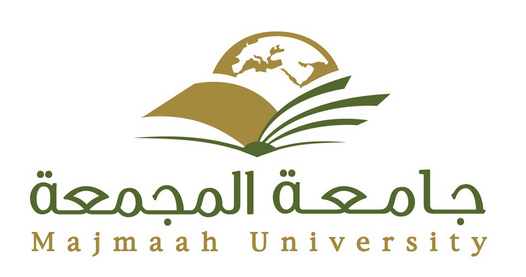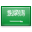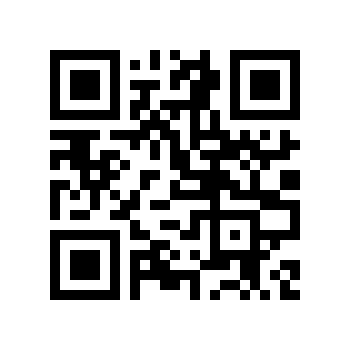# Shell integrationA volume is approximated by a collection of hollow cylinders. As the cylinder walls get thinner the approximation gets better. The limit of this approximation is the shell integral.

Shell integration (the shell method in integral calculus) is a means of calculating the volume of a solid of revolution, when integrating along an axis perpendicular to the axis of revolution. This is in contrast to disc integration which integrates along the axis parallel to the axis of revolution.

## Definition

The shell method goes as follows: Consider a volume in three dimensions obtained by rotating a cross-section in the xy-plane around the y-axis. Suppose the cross-section is defined by the graph of the positive function f(x) on the interval [ab]. Then the formula for the volume will be:

${displaystyle 2pi int _{a}^{b}xf(x),dx}$If the function is of the y coordinate and the axis of rotation is the x-axis then the formula becomes:

${displaystyle 2pi int _{a}^{b}yf(y),dy}$If the function is rotating around the line x = h or y = k, the formulas become:

${displaystyle {egin{cases}displaystyle 2pi int _{a}^{b}(x-h)f(x),dx,&{ ext{if}} hleq aand

${displaystyle {egin{cases}displaystyle 2pi int _{a}^{b}(y-k)f(y),dy,&{ ext{if}} kleq aThe formula is derived by computing the double integral in polar coordinates.

## Example

Consider the volume, depicted below, whose cross section on the interval [1, 2] is defined by:

${displaystyle y=(x-1)^{2}(x-2)^{2}}$In the case of disk integration we would need to solve for x given y. Because the volume is hollow in the middle we will find two functions, one that defines the inner solid and one that defines the outer solid. After integrating these two functions with the disk method we subtract them to yield the desired volume.

With the shell method all we need is the following formula:

${displaystyle 2pi int _{1}^{2}x(x-1)^{2}(x-2)^{2},dx}$By expanding the polynomial the integral becomes very simple. In the end we find the volume is π/10 cubic units.

### أهلاً ومرحباً بكم## قسم الرياضيات### التوقيت والتقويم

```_time.start(["thetimenow"]);
```

```
```

```

```

### محرك بحث جوجل```

```

### للتواصل1. الهاتف : 0164044771

تحويلة: 4771

(QR Code)

mailto:mm.mousa@mu.edu.sa### إعلانات1- الاختبار الفصلى الثانى لمقرر التحليل العددى (يوم الاحد الموافق 3 / 7/ 1440 هـ)

2- الاختبار الفصلى الثانى لمقررحساب المتجهات (يوم الثلاثاء الموافق 5 / 7 / 1440 هـ)

### الساعات المكتبيةالأثنين: 10 - 12

الثلاثاء: 8 - 10

الأربعاء: 8 - 10

### بعض الخدمات الإلكترونية لعمادة تقنية المعلومات### آلة حاسبة

```

```

### التقويم الجامعى### بعض الجوائز والتكريمات### إحصائية الموقع

عدد الصفحات: 258

البحوث والمحاضرات: 155

الزيارات: 102197